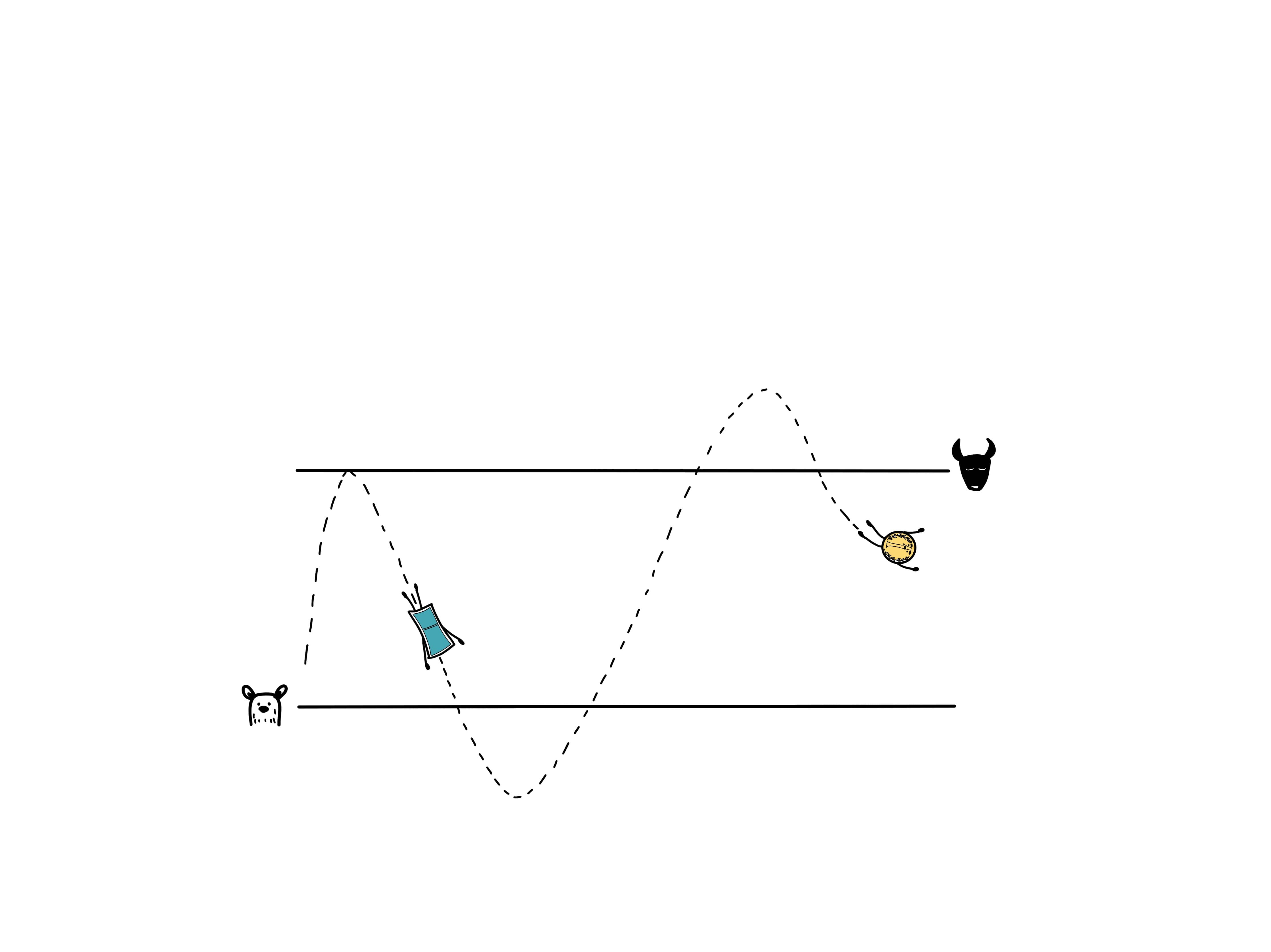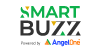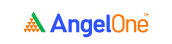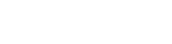# What is Relative Strength Index?

4.8Stock market trading has evolved with the advent of computers and advanced charting tools. Even a simple mobile application can provide you with graphs and charts that would be only available to large traders a few years ago. The availability of advanced metrics and indicators will be of limited use if the broader context and nuances are not understood. A major confusion for many traders and investors is the difference between relative strength and RSI or relative strength index. Both the metrics have similar-sounding names which is a major factor contributing to the prevailing confusion. To know relative strength vs relative strength index, you will have to understand both the indicators.

Relative Strength Index (RSI) is one of the most extensively used momentum oscillators in the realm of technical analysis of stocks. It was introduced by Welles Wilder in June 1978 and its computation is explained in detail in his book New Concepts in Technical Trading System. Momentum oscillator measures the velocity and magnitude of price movements of a security. RSI compares the magnitude of average gains and average losses of a security for drawing inferences about its strength and weakness over a predetermined time period.

## Relative strength

The relative strength is a technique that compares the value of a security to another security, index or benchmark. The relative strength can be considered to be part of the value investing system. The relative strength is represented by a ratio. It is derived by dividing the base security by the security, index or benchmark which is to be used for the comparison. If the benchmark index like BSE Sensex is to be used for comparison, you will have to divide the current price of the security with the level of the Sensex. Another stock of the same sector or a sectoral index can also be used to derive the relative strength. In the case of relative strength comparison between peers, it is important to compare stocks that have a strong historical correlation.

## Relative strength index

The relative strength index or RSI is a technical tool used in momentum investing. The RSI is represented as an oscillator, which is a line graph with two extremes. The RSI has a value between 0 and 100, which is calculated by taking recent price movements into consideration. An RSI value of over 70 is a signal of the stock being in the overbought territory and is hence overvalued, while a value lower than 30 is a signal of the stock being in the oversold territory and is therefore undervalued. To take action based on the RSI, investors should take another indicator into consideration to confirm the prevailing trend.

### Difference in calculations

A relative strength comparison can be done simply by dividing the price of the base security with the value of the reference index or security. For instance, suppose you have to do a relative strength comparison of stock ABC with the benchmark index BSE Sensex. Just divide the current market price of ABC with the current level of the benchmark.  If the price of ABC is Rs 1000 and Sensex is at 30,000, the relative strength of ABC will be 0.033.

A major difference between relative strength and RSI is the method of the calculation. While the relative strength can be calculated easily, the calculation of the relative strength index is slightly complicated. It has to be computed in a two-step calculation.

RSI step one = 100 – [100/ 1+ average gain/average loss]

Generally, the value of 14 periods is used for the calculation of the initial RSI. After data from 14 intervals is calculated, the second level of the RSI formula can be used.

RSI step two = 100 – [100/ 1 + (previous avg. gain*13+current gain)/(previous avg. loss *13+current loss)]

The formula will give the value of RSI, which is generally plotted below the price chart of the stock. The second formula smoothens the result and hence the value will near 0 or 100 only during strong trends.

### Usage

The utility of both indicators is another factor in relative strength vs RSI. The RSI is a momentum indicator which tells if security is oversold or overbought. For example, when the RSI is in the oversold territory and forms a higher low which matches with a corresponding low in the price of the stock, it is a signal of a bullish divergence. In such a situation any break above the oversold line can be used to take a long position.

In the case of relative strength, the historical value has to be taken to take action. If the relative strength ratio is lower than the historical value, investors can take a long position in the base security and short position in comparative security.

### Limitations of the RSI

The RSI compares bullish and bearish price momentum and displays the results in an oscillator that can be placed beneath a price chart. Like most technical indicators, its signals are most reliable when they conform to the long-term trend.

True reversal signals are rare and can be difficult to separate from false alarms. A false positive, for example, would be a bullish crossover followed by a sudden decline in a stock. A false negative would be a situation where there is a bearish crossover, yet the stock accelerated suddenly upward.

Since the indicator displays momentum, it can stay overbought or oversold for a long time when an asset has significant momentum in either direction. Therefore, the RSI is most useful in an oscillating market where the asset price is alternating between bullish and bearish movements.

#### Wrapping up

The difference between relative strength and RSI is essentially a difference of perspective. The relative strength tells about the value of a stock in comparison to another stock, index or benchmark, while the RSI tells about the performance of a stock in comparison to the recent performance of the same stock.

#### A Quick Recap

• The relative strength index (RSI) is a popular momentum oscillator developed in 1978.
• The RSI provides technical traders signals about bullish and bearish price momentum, and it is often plotted beneath the graph of an asset's price.
• An asset is usually considered overbought when the RSI is above 70% and oversold when it is below 30%.Take the quiz for this chapter & mark it complete.

How would you rate this chapter?

Get Information Mindfulness!

Catch-up With Market

News in 60 Seconds.The perfect starter to begin and stay tuned with your learning journey anytime and anywhere.